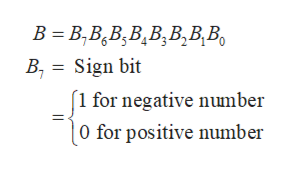Question
1 views

what is -4 (negative 4) in a 2's complement of 8 bits?

check_circle

Step 1

8-bit representation of a binary number is extremely useful and it is often called sign-magnitude representation of binary numbers. In this case, the left most bit is called the sign bit and it is used to represent whether the number is positive or negative. The remaining 7 bits are called magnitude bits and they represents the magnitude of the binary number. It is illustrated below for a 8-bit binary number B:help_outlineImage TranscriptioncloseB = B,B,B;B,B,B,B,B, B, = Sign bit (1 for negative number |0 for positive number fullscreen
Step 2

So, the 8-bit binary representation of the number +4 will be as follows:

Step 3

Now, 1’s complement of a binary number can be obtained by replacing all the 1’s by 0’s and all the 0’s by 1’s. So, the 1’s complem...

### Want to see the full answer?

See Solution

#### Want to see this answer and more?

Solutions are written by subject experts who are available 24/7. Questions are typically answered within 1 hour.*

See Solution
*Response times may vary by subject and question.
Tagged in

### Electrical Engineering